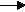gcsescience.com                                       4                                       gcsescience.com

The Reaction of Metals with Dilute Acid.

Potassium, sodium, lithium and calcium all react violently
with dilute sulfuric acid and dilute hydrochloric acid.
It is dangerous to put these metals into an acid.
The reaction is similar to the reaction with water,
forming the metal salt (either sulfate or chloride) plus H2(g).

For example

sodium + hydrochloric acidsodium chloride  +   hydrogen.
2Na(s)       +     2HCl(aq)2NaCl(aq)      +      H2(g)

Magnesium, aluminium, zinc, iron, tin and lead
react safely with dilute acid.
Magnesium is the fastest and lead is the slowest of the six.

magnesium + sulfuric acidmagnesium sulfate + hydrogen.
Mg(s)    +    H2SO4(aq)MgSO4(aq)     +         H2(g)

magnesium + hydrochloric acidmagnesium chloride + hydrogen.
Mg(s)       +   2HCl(aq)MgCl2(aq)      +      H2(g)
(see how to measure the rate of the reaction)

aluminium + hydrochloric acidaluminium chloride +  hydrogen.
2Al(s)       +       6HCl(aq)2AlCl3(aq)      +      3H2(g)

zinc   sulfuric acidzinc sulfate   +   hydrogen.
Zn(s)  +  H2SO4(aq)ZnSO4(aq)      +        H2(g)

iron + hydrochloric acidiron(II) chloride     +   hydrogen.
Fe(s)    +    2HCl(aq)FeCl2(aq)         +         H2(g)

tin + hydrochloric acidtin(II) chloride  +   hydrogen.
Sn(s)    +    2HCl(aq)SnCl2(aq)         +        H2(g)

lead  sulfuric acidlead sulfate   +   hydrogen.
Pb(s)  +  H2SO4(aq)PbSO4(s)      +        H2(g)

The reaction of zinc with sulfuric acid is often used to
make a small amount of hydrogen in the laboratory - see moles
.
The reaction is slow at room temperature
but its rate can be increased by the
addition of a little copper(II) sulphate.
Zinc displaces copper metal, which acts as a catalyst.

Metals below hydrogen in the reactivity series
(copper, silver, gold and platinum) will not react with dilute acid.
They cannot displace hydrogen from the non-metal anion.
See displacement reactions (next page).

gcsescience.com    The Periodic Table    Index    Reactivity Series Quiz    gcsescience.com